# SSC Quantitative Aptitude Questions (Day-69)

Dear Aspirants, you can find the Quantitative Aptitude questions with detailed explanations for the SSC exams. Nowadays the competitive level of the exam has been increasing consistently. Due to the great demand for the government job, the level of the toughness reached greater. Candidates have to enhance the preparation process in order to drive in the right path. It doesn’t need to clear the prescribed cutoff. You must have to score good marks more than the cut off marks to get into the final provisional list. Here we have updating the Quantitative Aptitude questions with detailed explanations on a daily basis. You can practice with us and measure your level of preparation. According to that you can sculpt yourself in a proper way. SSC aspirants kindly make use of it and grab your success in your career.

Start Quiz

1) If x = 7 + 210, then what is the value of √x1/√x ?

(a) 2√2

(b) 2/3 (2√5+√2)

(c) –2√2

(d) 2/3 (2√2+√5)

2) Which of the following relation(s) is/are true?

I) √7 + √3 > √5 + √5

II) √5 + √5 > √2 + √8

III) √5 + √5 > √7 + √3

(a) Only I

(b) Only II and III

(c) Only I and III

(d) All I, II and  III

3) If a = 1 + √3, b = 1 – √3 , then what is the value of a2 + b2 ?

(a) 4

(b) 8

(c) 0

(d) 2

4) What is the unit’s place of 12123?

(a) 2

(b) 4

(c) 6

(d) 8

5) How many two digit numbers are divisible by 3 but not by 7?

(a) 13

(b) 26

(c) 30

(d) 47

6) Pipe A can fill a tank in 12 hours and Pipe B can fill the tank in 18 hours. If both the pipes are opened on alternate hours and if pipe B is opened first,then in how much time (in hours) the tank will be full?

(a) 14 1/3

(b) 17 1/3

(c) 14 1/2

(d) 16 1/2

7) A, B and C can do a piece of work in 20, 24 and 30 days respectively. All three of them began the work together but B left 3 days before completion ofthe work. In how many days was the work completed?

(a) 7

(b) 10

(c) 12

(d) 9

8) The marked price of an article is Rs 8480. If a discount of 12.5% is given, then what will be the selling price (in Rs) of the article?

(a) 7420

(b) 6890

(c) 6360

(d) 7380

9) An item is sold for Rs 1428 after two successive discounts of 12.5% and 20%. What is the marked price (in Rs) of the article?

(a) 2000

(b) 2100

(c) 2040

(d) 2400

10) If
A/B = 2/3, B/C = 4/5 and  C/D = 3/2, then what is the ratio of A : B : C : D?

(a) 8 : 12  : 10 : 15

(b) 8 : 15 : 12 : 10

(c) 8 : 10 : 12 : 15

(d) 8 : 12 : 15 : 10

x = (√5 + √2)2

√x = √5 + √2

1/√x = (√5 – √2) / 3

= 1/3 (√5 – √2)

√x – 1/√x = (√5 + √2) – 1/3 (√5 – √2)

= 2/3 √5 + 4/3 √2

= 2/3 (√5 + 2√2)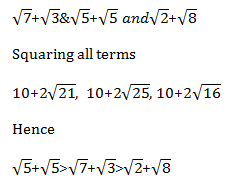a = 1 + √3

b = 1 – √3

a² = 1 + 3 + 2√3

= 4 + 2√3

b² = 4 – 2√3

a² + b² = 8

12¹²³⇒

We know that unit’s digitis  having cyclicity as 4.

2¹ = 2

2² = 4

2³ = 8

2⁴ = 6

123 = 4K + 3

Hence for 12¹²³ last digit ⇒ 8

No. of two digit  Nos. divisible by 3 below 100 = 33-3 =30

No. of Nos. divisible by 3 & 7 both or multiple of 21,

= 100/21 = 4 Nos.

Hence total nos. divisible by 3 but not 7

= 30 – 4

= 26 Nos.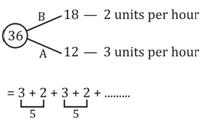In 14 days it will work 35 units on last day B will work for ½ day.

[1 unit work on 15th day]

Hence ⇒ 14 ½ days.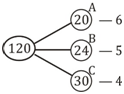In 3 days B will work 5 × 3 = 15 unit work

New work ⇒ 135

Total days required to do the work

= 135/15

= 9 days

12.5% = ⅛ of given price

Discount =  8480/8 = 1060

S.P. = M.P. – disc. = 7420/-

New price after successive disc will be ,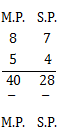∵28 → 1428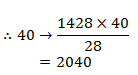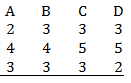24  :  36   :  45   :  30

8 : 12 : 15 : 10

 Check Here to View SSC CGL / CHSL 2021 Quantitative Aptitude Questions Day – 68 Day – 67 Day – 66